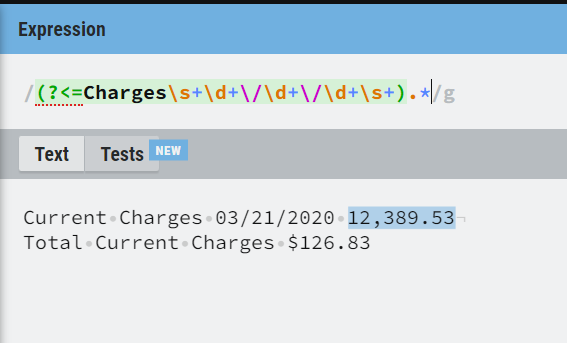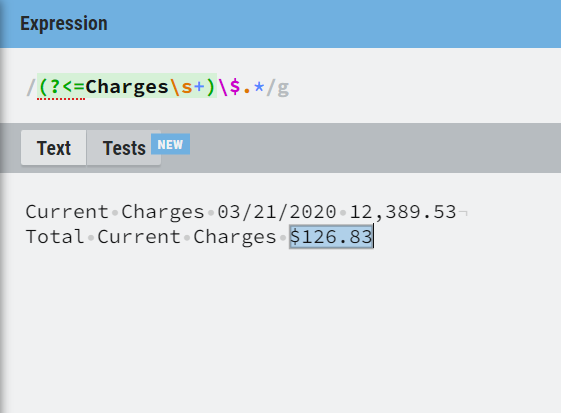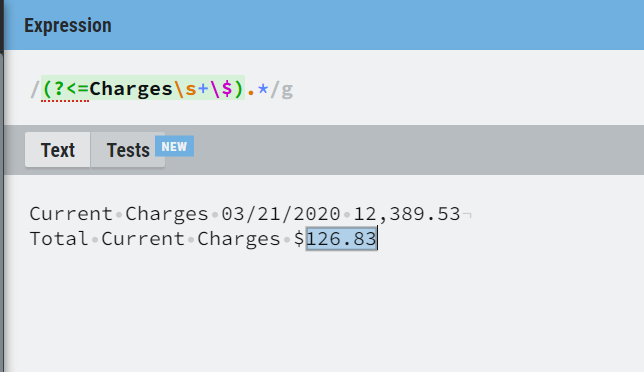# Regex help need

Hi, Trying the following regex just to get amount. It is also getting mm from month. Can you help? Thanks a lot,

(?<=Current Charges\s*(\d{1,2}/\d{1,2}/\d{4}\s+)?)\s?\$?[\s?\d.,]+

Current Charges 03/21/2020 12,389.53
Total Current Charges \$126.83

1 Like

@A_Learner

Use below regex

``````System.Text.RegularExpressions.Regex.Matches(yourinputvariable.ToString,"((\d+\,?\d+\.\d+)")
``````

Or Use the below if the dollar symbol also required

``````System.Text.RegularExpressions.Regex.Matches(yourinputvariable.ToString,"((((?<=Current Charges\s+(\d+\/\d+\/\d+\s+)?)\\$?\d+\,?\d+\.?\d+))")
``````

`(?<=Current Charges\s*\d{1,2}/\d{1,2}/\d{4}\s+).*?([\d,.]+)`

Regards,

Use the below regex expression

To extract the current charge only

``````System.Text.RegularExpressions.Regex.Matches(yourstringinput.ToString,“((?<=Charges\s+\d+\/\d+\/+\d+\s+).*)”)
``````To extract the Total current charges with \$

``````System.Text.RegularExpressions.Regex.Matches(yourstringinput.ToString,“((?<=Charges\s+)\\$.*)”)
``````To extract the Total Current Charges with out \$

``````System.Text.RegularExpressions.Regex.Matches(yourstringinput.ToString,“((?<=Charges\s+\\$).*)”)
``````To extract the both values

``````System.Text.RegularExpressions.Regex.Matches(yourstringinput.ToString,“((?<=Charges\s+\d+\/\d+\/\d+\s+).*|(?<=Charges\s+)\\$.*)”)
``````

Hope it helps!!

1 Like
1. (?<=Total).*
2. .*(?=Total Current Charges)

Both of this approach should work

Thanks a lot. It fits my requirement @mkankatala

Thanks every one for your help.

Thank you @Ritaman_Baral

Happy Automation!!

This topic was automatically closed 3 days after the last reply. New replies are no longer allowed.